﻿ 飞秒激光成丝对微波传输的影响研究

# 飞秒激光成丝对微波传输的影响研究Influence of Femtosecond Filaments on Microwave Propagation

Abstract: The effects of femtosecond filaments on microwave transmission are studied by experiments and numerical simulations, including the influences of incident angle of the microwave and separation distance of filaments on microwave reflection coefficient. The results indicate that when the plane of the incident microwave is perpendicular to the propagation direction of the filaments, the reflection coefficient increases with the increase of the incident angle of S-polarized microwave. When the plane of the P-polarized incident microwave is parallel to the filaments, the reflection coefficient decreases as the incident angle increases. Besides, the reflection will be enhanced by reducing the separation distance between the filaments.

1. 引言

2. 数值仿真研究

2.1. 模型设计

${\eta }_{f}={\left(1-\frac{{\omega }_{pe}^{2}}{{\omega }^{2}\left(1+i{v}_{e}/\omega \right)}\right)}^{1/2}$ (1)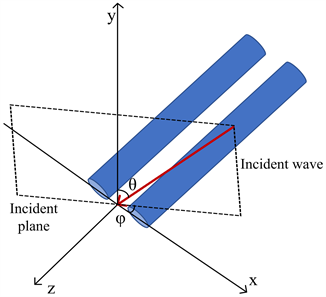Figure 1. Geometry used to study the interaction of the incident microwave with the femtosecond filaments

2.2. 数值仿真结果与分析

2.2.1. 微波入射角度对反射率的影响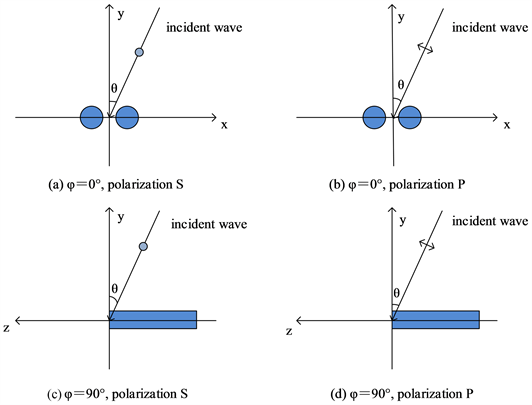Figure 2. Geometry of the polarization direction of microwave in numerical calculation: (a) φ = 0˚, Polarization S, (b) φ = 0˚, Polarization P and (c) φ = 90˚, Polarization S, and (d) φ = 90˚, Polarization P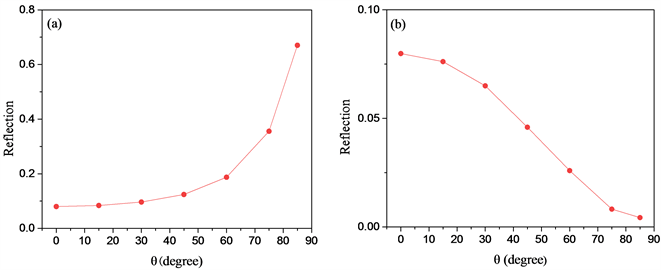Figure 3. Influence of the incident angle of microwave on the reflection coefficient under the conditions of (a) φ = 0˚, polarization S and (b) φ = 90˚, polarization P, respectively

2.2.2. 等离子体丝间距对反射率的影响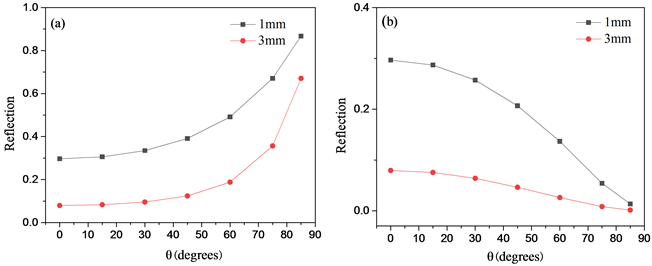Figure 4. Influence of the spacing of the filaments on the reflection coefficient for (a) φ = 0˚, polarization S and (b) φ = 90˚, polarization P incident microwave respectively

3. 实验部分

3.1. 实验装置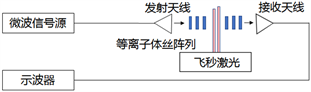Figure 5. Experimental setup

3.2. 结果与讨论

3.2.1. 微波入射角度对反射率和吸收率的影响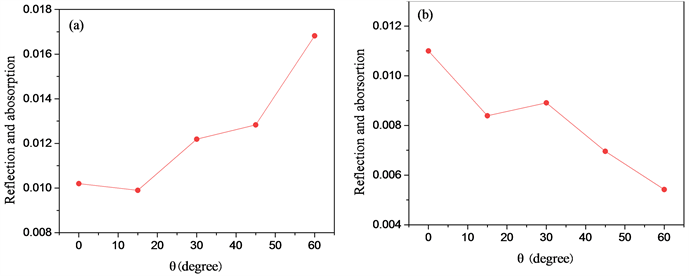Figure 6. Influence of the incident angle of microwave on the sum of reflection and absorption coefficients under the conditions of (a) φ = 0˚, polarization S and (b) φ = 90˚, polarization P, respectively

3.2.2. 等离子体丝间距对反射率和吸收率的影响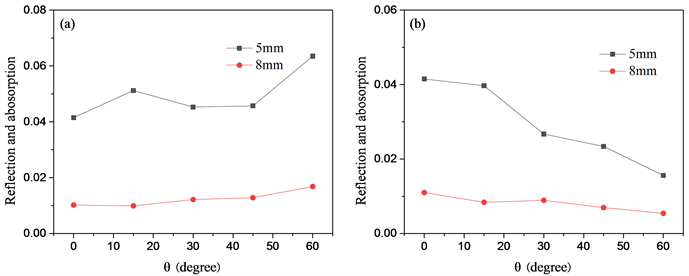Figure 7. Influence of the spacing of the filaments on the sum of reflection and absorption coefficients for (a) φ = 0˚, polarization S and (b) φ = 90˚, polarization P microwave, respectively

4. 结论

NOTES

*通讯作者。

 Wöste, L., Wedekind, C., Wille, H., Rairoux, P., Stein, B., Nikolov, S., Werner, C., Niedermeier, S., Ronnenberger, F., Schillinger, H. and Sauerbrey, R. (1997) Femtosecond Atmospheric Lamp. Laser and Optoelektron, 29, 51-53.

 Hao, Z.Q., Zhang, J., Yu, J., Zheng, Z.Y., Yuan, X.H., Zhang, Z., Li, Y.T., Wang, Z.H., Ling, W.J. and Wei, Z.Y. (2006) The Comparison Study of Diagnostics of Light Filaments in Air. Science in China: G, 49, 228-235.
https://doi.org/10.1007/s11433-006-0228-7

 Bogatskaya, A.V., Popov, A.M. and Smetanin, I.V. (2014) Amplification and Guiding of Microwave Radiation in a Plasma Channel Created by an Ultrashort High-Intensity Laser Pulse in Noble Gases. Journal of Russian Laser Research, 35, 437-446.
https://doi.org/10.1007/s10946-014-9445-0

 赵朋程, 郭立新, 李慧敏. 110 GHz高功率微波在大气击穿等离子体中的传输、反射和吸收[J]. 电波科学学报, 2016, 31(3): 512-515.

 Alshershby, M., Lin, J.Q. and Hao, Z.Q. (2012) Numerical Analysis of Guiding a Microwave Radiation Using a Set of Plasma Filaments: Dielectric Waveguide Concept. Applied Physics B, 45, 065102.
https://doi.org/10.1088/0022-3727/45/6/065102

 Naidis, G.V. (2001) On Propagation Characteristics of Electromagnetic Waves along a Plasma Filament. Journal of Physics D, 34, L103-L104.
https://doi.org/10.1088/0022-3727/34/18/101

 Musin, R.R., Shneider, M.N., Zheltikov, A.M. and Miles, R.B. (2007) Guiding Radar Signals by Arrays of Laser-Induced Filaments: Finite-Difference Analysis. Applied Optics, 46, 5593-5597.
https://doi.org/10.1364/AO.46.005593

 Alshershby, M., Hao, Z.Q. and Lin, J.Q. (2012) Guiding Microwave Radiation Using Laser-induced Filaments: The Hollow Conducting Waveguide Concept. Journal of Physics D, 45, 265401.
https://doi.org/10.1088/0022-3727/45/26/265401

 Liu, Y., Chen, Z.S. and Shi, J.M. (2019) Simulation of HPM Propagation in a Double-Line Containing Plasma Filaments Produced by Intense Femto Second Laser Pulses. IEEE Transactions on Plasma Science, 47, 1394-1398.
https://doi.org/10.1109/TPS.2018.2887231

 Marian, A., Morsli, M.E., Vidal, F. and Payeur, S. (2013) The Interaction of Polarized Microwaves with Planar Arrays of Femto Second Laser-produced Plasma Filaments in Air. Physics of Plasmas, 20, 023301.
https://doi.org/10.1063/1.4792160

 朱安石, 陈自力, 杨勇, 刘晓倩, 甄云卉. 等离子体与电磁波相互作用FDTD数值模拟[J]. 现代雷达, 2015, 37(8): 72-80.

 刘洋, 程立, 汪家春, 王启超, 袁忠才, 时家明. 发光等离子体对6 GHz高功率微波的防护性能研究[J]. 发光学报, 2016, 37(10): 1292-1298.

 孙中浩, 董超, 张亚春, 何湘, 倪晓武, 骆晓森. 飞秒光丝阵列对10 GHz电磁波的吸收特性[J]. 强激光与粒子束, 2018, 30(5): 053201.

 李志刚, 程立, 马志伟, 汪家春, 时家明. 入射频率对高功率微波与等离子体相互作用的影响分析[J]. 国防科技大学学报, 2018, 40(4): 47-52.

 Huba, J.D. (2011) NRL Plasma Formulary. Naval Research Laboratory, Washington DC.

 吴莹. 激光等离子体的微波干扰和诊断研究[D]: [博士学位论文]. 南京: 南京理工大学, 2009.

 FF•陈. 等离子体物理学导论[M]. 林光海, 译. 北京: 科学出版社, 2016.

 董超, 孙中浩, 张亚春, 何湘, 倪晓武, 骆晓森. 激光等离子体丝阵列对10GHz微波传输特性的影响[J]. 红外与激光工程, 2018, 47(10): 160-167.

Top